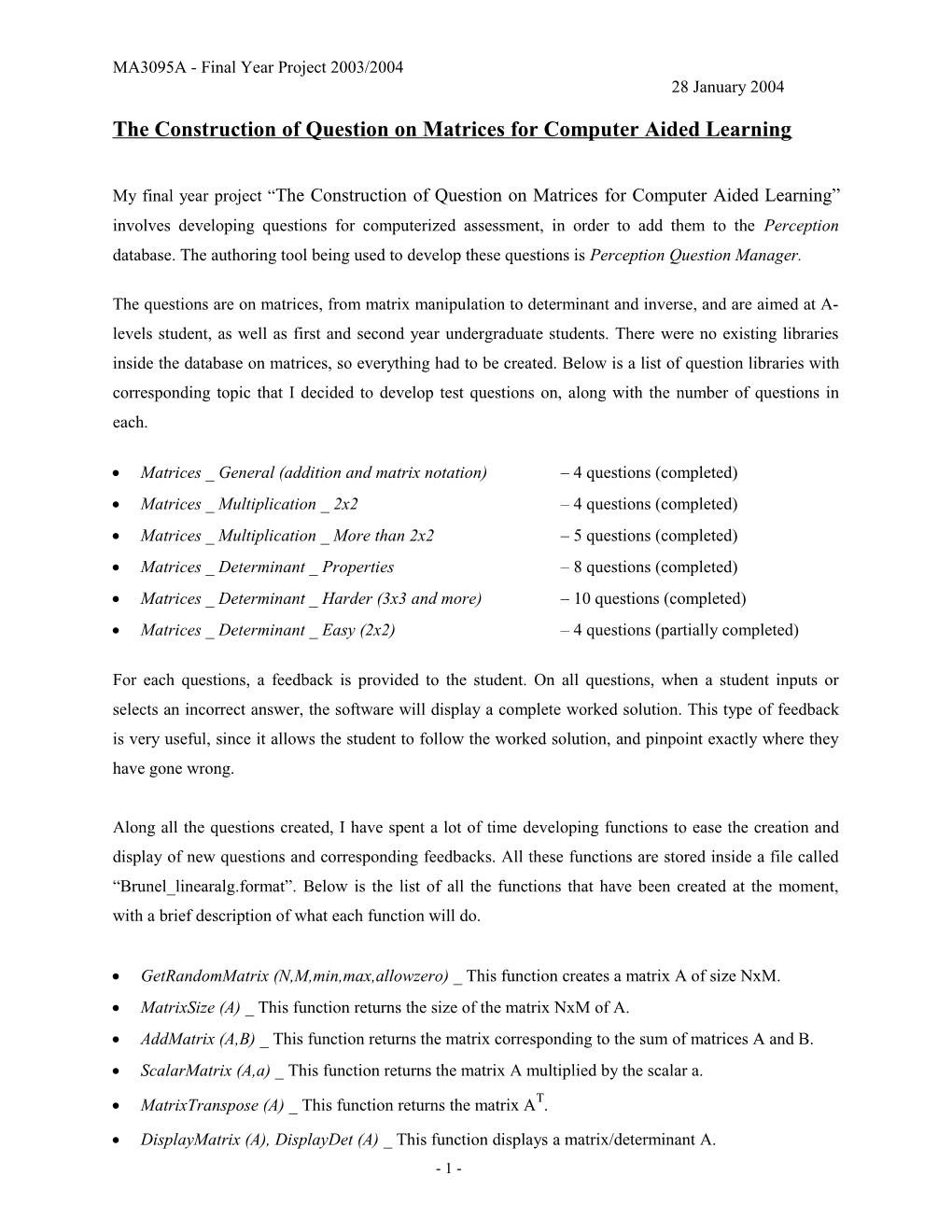# The Construction of Question on Matrices for Computer Aided LearningMA3095A - Final Year Project 2003/2004

28 January 2004

### The Construction of Question on Matrices for Computer Aided Learning

My final year project “The Construction of Question on Matrices for Computer Aided Learning” involves developing questions for computerized assessment, in order to add them to the Perception database. The authoring tool being used to develop these questions is PerceptionQuestion Manager.

The questions are on matrices, from matrix manipulation to determinant and inverse, and are aimed at A-levels student, as well as first and second year undergraduate students. There were no existing libraries inside the database on matrices, so everything had to be created. Below is a list of question libraries with corresponding topic that I decided to develop test questions on, along with the number of questions in each.

• Matrices _ General (addition and matrix notation)– 4 questions (completed)
• Matrices _ Multiplication _ 2x2– 4 questions (completed)
• Matrices _ Multiplication _ More than 2x2– 5 questions (completed)
• Matrices _ Determinant _ Properties– 8 questions (completed)
• Matrices _ Determinant _ Harder (3x3 and more)– 10 questions (completed)
• Matrices _ Determinant _ Easy (2x2)– 4 questions (partially completed)

For each questions, a feedback is provided to the student. On all questions, when a student inputs or selects an incorrect answer, the software will display a complete worked solution. This type of feedback is very useful, since it allows the student to follow the worked solution, and pinpoint exactly where they have gone wrong.

Along all the questions created, I have spent a lot of time developing functions to ease the creation and display of new questions and corresponding feedbacks. All these functions are stored inside a file called “Brunel_linearalg.format”. Below is the list of all the functions that have been created at the moment, with a brief description of what each function will do.

• GetRandomMatrix (N,M,min,max,allowzero) _ This function creates a matrix A of size NxM.
• MatrixSize (A) _ This function returns the size of the matrix NxM of A.
• AddMatrix (A,B) _ This function returns the matrix corresponding to the sum of matrices A and B.
• ScalarMatrix (A,a) _ This function returns the matrix A multiplied by the scalar a.
• MatrixTranspose (A) _ This function returns the matrix AT.
• DisplayMatrix (A), DisplayDet (A) _ This function displays a matrix/determinant A.
• DisplayMatrix_Coloured (A,a,b) _ This function displays a matrix A with either one element Aa,b, either the row a, either the column b or either row a and column b colored with a defined background and font color.
• MultiMatrix (A,B) _ This function calculates the matrix AB.
• PowerMatrix (A,n) _ This function calculates the matrix An.
• DoMinnor (A,a,b) _ This function returns the minor matrix of A at the entry A a,b.
• Determinant (A) _ This function calculates the determinant of any matrix A of size NxN.
• Cofactors (A,a,b) _ This function calculates the cofactor of the matrix A at the entry A a,b.

The next step in my project will be to produce questions on inverse matrices:

-asking the student to find the inverse of a given matrix A in a Multiple-Choice question,

-asking the student to find the matrix A given the inverse in a Multiple-Choice question,

-or asking to find one element of the Inverse matrix by a Numerical Input question.

For that I intend to create also new functions such as an Inverse (A) that will return the inverse of the matrix A, and also displayInverse (A) that will display the inverse of A in the form Adj(A).

The Gantt chart below shows what remains to be done for my final project and the estimated time allocation. It includes the 3 main chapters left of my write up (Chapter 2 and 5 being completed, and Chapter being in good progress of completion).

4th-Feb / 11th-Feb / 18th-Feb / 25th-Feb / 3rd-Mar / 10th-Mar / 17th-Mar / 24th-Mar
Complete "determinant" library
Create "inverse" library
Create functions for "inverse"
Testing
Complete "Chapter 3 on Determinant"
Write Up and Complete "Chapter 4 on Inverse"
Write Up and Complete "Chapter 1 on CAA"
Write Up Introduction, Conclusion and Recommendations

- 1 -